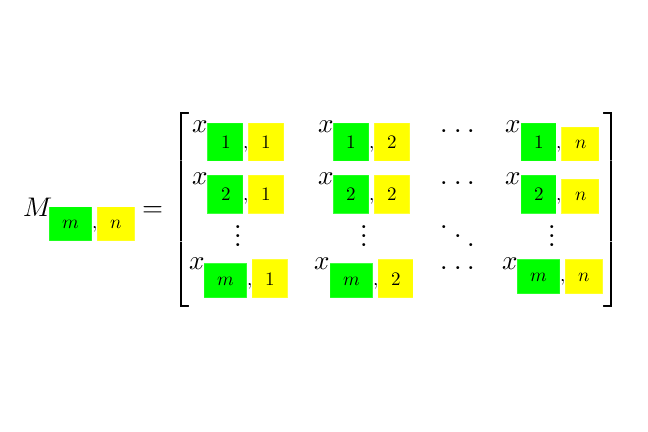## Want to keep learning?

This content is taken from the UNSW Sydney's online course, Maths for Humans: Inverse Relations and Power Laws. Join the course to learn more.
2.10

## UNSW SydneyAn m xn matrix

# Mobius composition and matrices

Composition of Mobius transformations has a particularly pleasant form, and connects naturally with the important topic of matrix multiplication from linear algebra.

In this step, you will

• learn how to multiply $$\normalsize 2\times 2$$ matrices

• see the connection between matrix multiplication and composition of Mobius functions.

## Mobius transformations and matrices

We have seen that a Mobius transformation, or fractional linear transformation, is a function of the form

$\Large{y=f(x)=\frac{ax+b}{cx+d}}$

where the numbers $$\normalsize{a}$$, $$\normalsize{b}$$, $$\normalsize{c}$$ and $$\normalsize{d}$$ can be arbitrary (subject to the common sense requirement that the fraction does not end up with a zero in the denominator!) So the function can be encoded by the matrix

$\Large{M = \begin{pmatrix} a & b\\ c & d \end{pmatrix}}.$

This kind of mathematical object is very important in linear algebra.

In this somewhat advanced step, you’ll see how the composition of fractional linear transformations connects directly with matrix multiplication.

Q1 (E): What is the corresponding matrix for the Mobius transformation

$\Large{f(x)=\frac{x}{3x+2}}?$

## How to multiply matrices

First of all here is how you multiply a row vector by a column vector (the three line $$\equiv$$ is used to signify a definition):

$\Large{\begin{pmatrix} a & b \end{pmatrix} \begin{pmatrix} e \\ g \end{pmatrix}\equiv \begin{pmatrix} ae+bg \end{pmatrix}}.$

When we multiply $$\normalsize 2\times 2$$ matrices, there are four possible operations of this kind, where we multiply the rows of the first matrix by the columns of the second matrix, and arrange the result in a matrix as follows:

$\Large{\begin{pmatrix} a & b\\\ c & d \end{pmatrix} \begin{pmatrix} e & f\\ g & h \end{pmatrix}\equiv \begin{pmatrix} ae+bg & af+bh\\ ce+dg & cf+ dh \end{pmatrix}}.$

So for example:

$\Large{\begin{pmatrix} 1 & 2\\ 1 & -1 \end{pmatrix} \begin{pmatrix} 2 & 1\\ 3 & 4 \end{pmatrix}= \begin{pmatrix} 8 & 9\\ -1 & -3 \end{pmatrix}.}$

Q2 (M): For the matrices

$\Large{A=\begin{pmatrix} 1 & 2\\ -1 & 5 \end{pmatrix}} \quad \text{and} \quad \Large{B=\begin{pmatrix} 3 & 1\\ 6 & 4 \end{pmatrix}}$

find the products $$\normalsize{AB}$$ and $$\normalsize{BA.}$$ Note that matrix multiplication is not in general commutative!

## Composition of Mobius transformations

Suppose we have two Mobius transformations

$\Large{k(x)=\frac{ax+b}{cx+d}} \quad \text{and} \quad {l(x)=\frac{ex+f}{gx+h}}.$

Then the composition $$\normalsize{k \circ l}$$ of these two transformations is

\Large{\begin{align} (k\circ l) (x) & = k(l(x)) \\ & =\frac{a\left(\frac{ex+f}{gx+h}\right)+b}{c\left(\frac{ex+f}{gx+h}\right)+d} \\ & =\frac{(a e+b g)x+(a f+b h)}{(c e + d g)x+ (c f+ d h)}.\end{align}}

This is exactly the Mobius transformation corresponding the matrix product

$\Large{ \begin{pmatrix} a & b\\ c & d \end{pmatrix} \begin{pmatrix} e & f\\ g & h \end{pmatrix} = \begin{pmatrix} ae+bg & af+bh\\ ce+dg & cf+ dh \end{pmatrix}}.$

We have demonstrated something interesting here. Composition of Mobius transformations corresponds exactly to multiplication of matrices!

Q3(M): Find the composition $$\normalsize{k \circ l}$$ of the Mobius transformations

$\Large{k(x)=\frac{x+1}{3x-2}}$

and

$\Large{l(x)=\frac{2x-1}{x}}.$

A1. The matrix for the Mobius transformation

$\Large{f(x)=\frac{x}{3x+2} \quad\text{is}\quad \begin{pmatrix} 1 & 0\\ 3 & 2 \end{pmatrix} }.$

A2. One product is

$\Large AB=\begin{pmatrix} 1 & 2\\ -1 & 5 \end{pmatrix}\begin{pmatrix} 3 & 1\\ 6 & 4 \end{pmatrix}= \begin{pmatrix} 1\times 3+ 2\times 6 & 1\times 1+2\times 4\\ -1\times 3+5\times 6 & -1\times 1+5\times 4 \end{pmatrix}=\begin{pmatrix} 15 & 9\\ 27 & 19 \end{pmatrix}.$

The other product is

$\Large BA= \begin{pmatrix} 3 & 1\\ 6 & 4 \end{pmatrix}\begin{pmatrix} 1 & 2\\ -1 & 5 \end{pmatrix}= \begin{pmatrix} 3\times 1+ 1\times (-1) & 3\times 2+1\times 5\\ 6\times 1+4\times (-1) & 6\times 2+4\times 5 \end{pmatrix}=\begin{pmatrix} 2 & 11\\ 2 & 32 \end{pmatrix}.$

So, $$\normalsize{AB\neq BA}$$!

A3. You could find this directly, but we’ll use matrix multiplication to solve this question. The matrix for the Mobius transformation

$\Large{k(x)=\frac{x+1}{3x-2} \quad \text{is} \quad \begin{pmatrix} 1 & 1\\ 3 & -2 \end{pmatrix} }.$

Similarly the matrix for the Mobius transformation

$\Large{l(x)=\frac{2x-1}{x} \quad \text{is}\quad \begin{pmatrix} 2 & -1\\ 1 & 0 \end{pmatrix} }.$

So, the matrix corresponding to the Mobius transformation

$\Large{ (k \circ l)(x) \quad \text{is}\quad \begin{pmatrix} 1 & 1\\ 3 & -2 \end{pmatrix}\begin{pmatrix} 2 & -1\\ 1 & 0 \end{pmatrix}=\begin{pmatrix} 3 & -1\\ 4 & -3 \end{pmatrix}}.$

Therefore, the composition is

$\Large{(k\circ l)(x) = \frac{3x-1}{4x-3}}.$